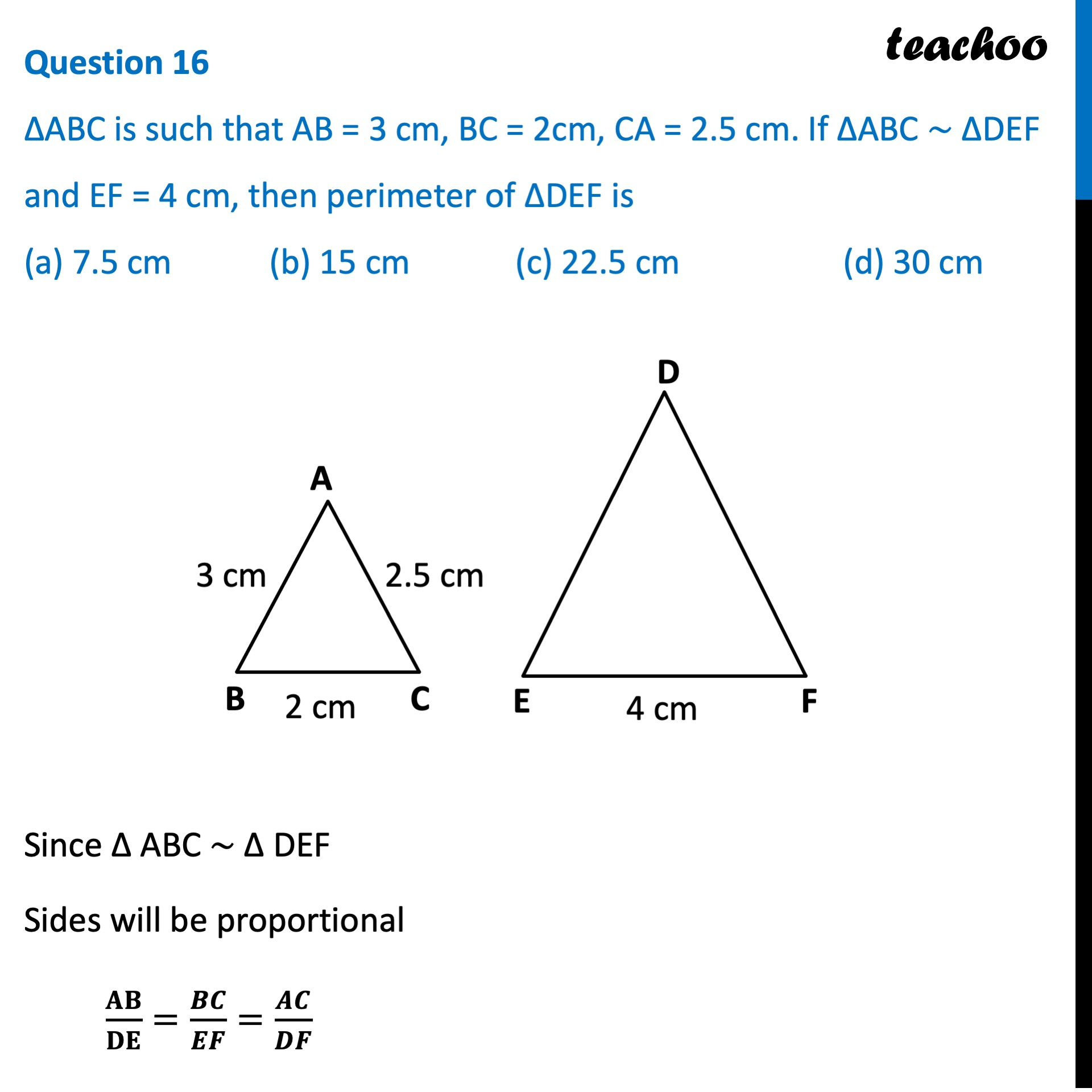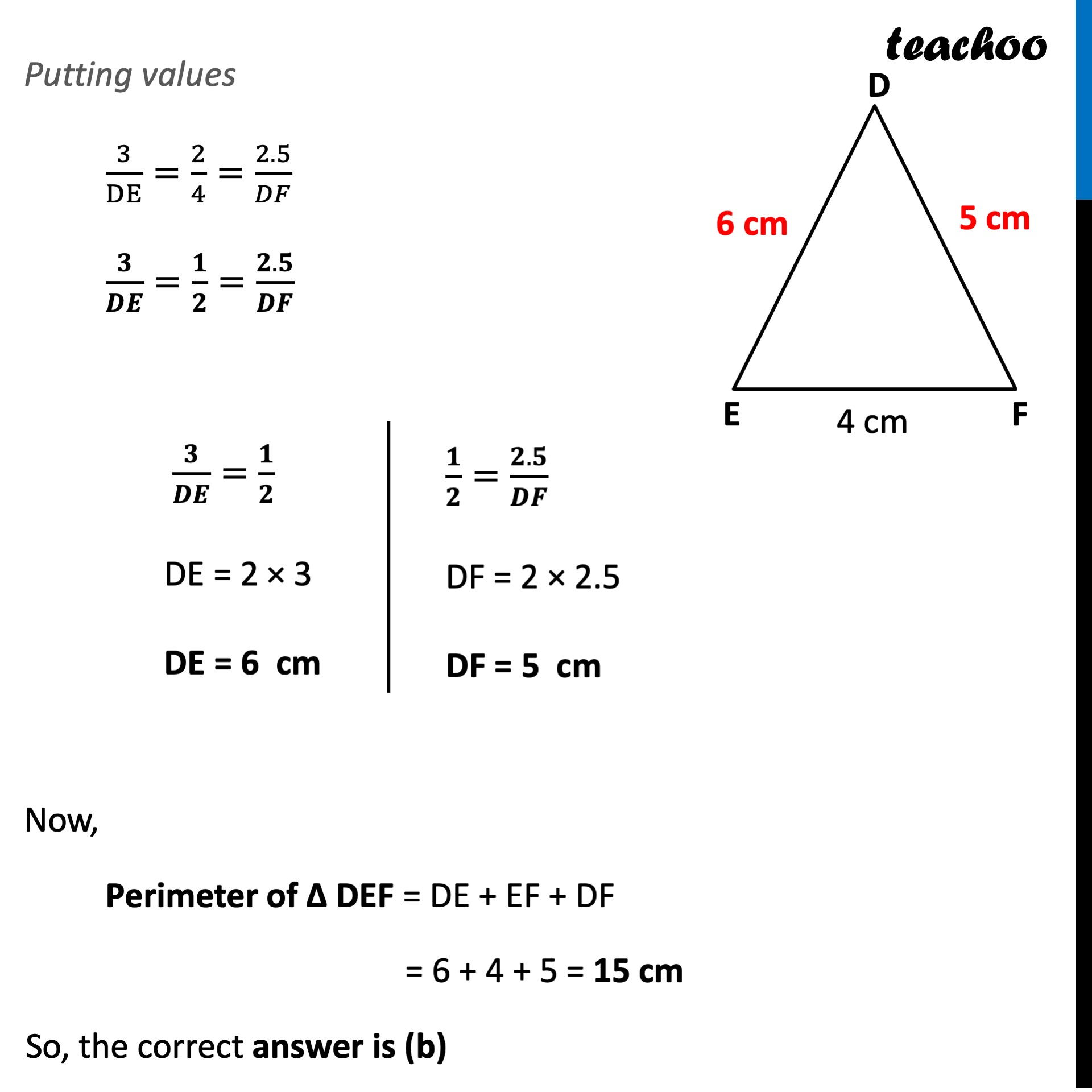CBSE Class 10 Sample Paper for 2022 Boards - Maths Standard [MCQ]

Class 10
Solutions of Sample Papers for Class 10 Boards

## (a) 7.5 cm   (b) 15 cm   (c) 22.5 cm   (d) 30 cmGet live Maths 1-on-1 Classs - Class 6 to 12

### Transcript

Question 16 ∆ABC is such that AB = 3 cm, BC = 2cm, CA = 2.5 cm. If ∆ABC ~ ∆DEF and EF = 4 cm, then perimeter of ∆DEF is (a) 7.5 cm (b) 15 cm (c) 22.5 cm (d) 30 cm Since ∆ ABC ~ ∆ DEF Sides will be proportional AB/DE=𝐵𝐶/𝐸𝐹=𝐴𝐶/𝐸𝐹 Putting values 3/DE=2/4=2.5/𝐸𝐹 3/DE=1/2=2.5/𝐸𝐹 𝟑/𝑫𝑬=𝟏/𝟐 DE = 2 × 3 DE = 6 cm 𝟏/𝟐=(𝟐.𝟓)/𝑬𝑭 EF = 2 × 2.5 EF = 5 cm Now, Perimeter of Δ DEF = DE + EF + DF = 6 + 5 + 4 = 15 cm So, the correct answer is (b)